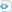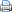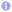C++

# C++11/14 for scientific computing III

## Mathematical functions

C++11/14 introduces several new mathematical functions, which are all overloaded for the types float, double and long double and that are defined in the header file cmath. The following table summerizes the new functions.

Basic operations
remainder signed remainder of the division operation
remquo signed remainder as well as the three last bits of the division operation
fmax larger of two floating point values
fmin smaller of two floating point values
fdim positive difference of two floating point values ($\max(0, x-y)$)
nan, nanf, nanl not-a-number (NaN)
##### Exponential functions
exp2 returns $2$ raised to the given power ($2^x$)
expm1 returns e raised to the given power, minus one ($\exp x -1$)
log2 base 2 logarithm of the given number ($\log_2x$)
log1p natural logarithm of 1 plus the given number ($\ln(1+x)$)
##### Power functions
cbrt computes cubic root ($\sqrt{x}$)
hypot computes square root of the sum of the squares of two given numbers ($\sqrt{x^2+y^2}$)
##### Hyperbolic functions
asinh computes the inverse hyperbolic sine
acosh computes the inverse hyperbolic cosine
atanh computes the inverse hyperbolic tangent
##### Error and gamma functions
erf error function
erfc complementary error function
tgamma gamma function
lgamma natural logarithm of the gamma function
##### Nearest integer floating point operations
trunc nearest integer not greater in magnitude than the given value
round, lround, llround nearest integer, rounding away from zero in halfway cases
nearbyint nearest integer using current rounding mode
rint, lrint, llrint nearest integer using current rounding mode with exception if the result differs
##### Floating point manipulation functions
scalbn, scalbln multiplies a number by FLT_RADIX raised to a power
ilogb extracts exponent of the number
logb extracts exponent of the number
nextafter, nexttoward next representable floating point value towards the given value
copysign copies the sign of a floating point value
##### Classification and comparison
fpclassify categorizes the given floating point value
isfinite checks if the given number has finite value
isinf checks if the given number is infinite
isnan checks if the given number is NaN
isnormal checks if the given number is normal
signbit checks if the given number is negative
isgreater checks if the first floating-point argument is greater than the second
isgreaterequal checks if the first floating-point argument is greater or equal than the second
isless checks if the first floating-point argument is less than the second
islessequal checks if the first floating-point argument is less or equal than the second
islessgreater checks if the first floating-point argument is less or greater than the second
isunordered checks if two floating-point values are unordered

## Minimum maximum functions

The header file algorithm defines template functions for determining the minimum or the maximum of two comparable objects of the same type, e.g., numbers. In C++11 overloaded versions of these functions have been introduced that allow to determine the minimum or the maximum of an arbitrary number of objects, which are passed via an initializer list. Furthermore, minmax determines both the minimum and the maximum in a single scan. The following code gives an illustrative example.

 Source code#include <iostream>
#include <algorithm>

int main() {
int i0(0), i1(1), i2(2), i3(3), i4(4);
// determine minimum and maximum seperately
std::cout << "min : " << std::min({i3, i1, i2, i0, i4}) << '\n'
<< "max : " << std::max({i3, i1, i2, i0, i4}) << '\n';
// determine minimum and maximum in a single scan
auto min_max=std::minmax({i3, i1, i2, i0, i4});
std::cout << "min : " << min_max.first << '\n'
<< "max : " << min_max.second << '\n';
}